# ISEE Upper Level Quantitative : Hexagons

## Example Questions

### Example Question #1 : Hexagons

Which is the greater quantity?

(a) The area of a regular hexagon with sidelength 1

(b) The area of an equilateral triangle with sidelength 2

(a) and (b) are equal

(a) is greater

(b) is greater

It is impossible to tell from the information given

(a) is greater

Explanation:

A regular hexagon with sidelength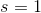can be seen as a composite of six equilateral triangles, each with sidelength. Since area is in direct proportion to the square of the sidelength, the area of the equilateral triangle with sidelength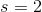is equal to that of four of those triangles. This makes the hexagon greater in area, and it makes (a) the greater quantity.

### Example Question #1 : Hexagons

Which is the greater quantity?

(a) The perimeter of a regular pentagon with sidelength 1 foot

(b) The perimeter of a regular hexagon with sidelength 10 inches

(b) is greater.

(a) is greater.

(a) and (b) are equal.

It is impossible to tell from the information given.

(a) and (b) are equal.

Explanation:

The sides of a regular polygon are congruent, so in each case, multiply the sidelength by the number of sides to get the perimeter.

(a) Since one foot equals twelve inches,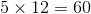inches.

(b) Multiply: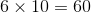inches

The two polygons have the same perimeter.

### Example Question #91 : Plane Geometry

A hexagon has six angles with measures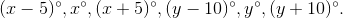Which quantity is greater?

(a)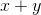(b) 240

It is impossible to tell from the information given

(a) is greater

(a) and (b) are equal

(b) is greater

(a) and (b) are equal

Explanation:

The angles of a hexagon measure a total of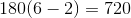. From the information, we know that: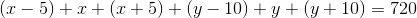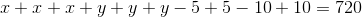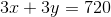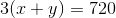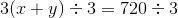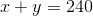The quantities are equal.

### Example Question #4 : Hexagons

A hexagon has six angles with measures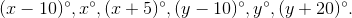Which quantity is greater?

(a)(b)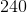(a) and (b) are equal.

It is impossible to tell from the information given.

(a) is greater.

(b) is greater.

(b) is greater.

Explanation:

The angles of a hexagon measure a total of.  From the information, we know that: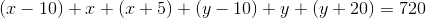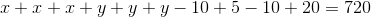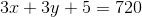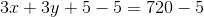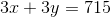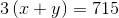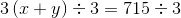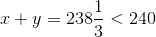This makes (b) greater.

### Example Question #5 : Hexagons

The angles of Hexagon A measure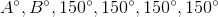The angles of Octagon B measure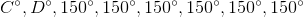Which is the greater quantity?

(A)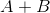(B)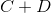(A) and (B) are equal

(B) is greater

(A) is greater

It is impossible to determine which is greater from the information given

(B) is greater

Explanation:

The sum of the measures of a hexagon is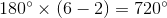. Therefore,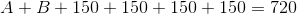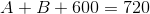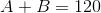The sum of the measures of an octagon is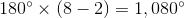. Therefore,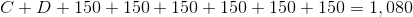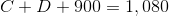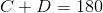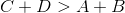, so (B) is greater.

### Example Question #6 : Hexagons

The angles of Pentagon A measure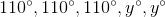The angles of Hexagon B measure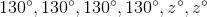Which is the greater quantity?

(A)(B)(A) and (B) are equal

(B) is greater

It is impossible to determine which is greater from the information given

(A) is greater

(A) is greater

Explanation:

The sum of the measures of the angles of a pentagon is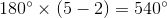. Therefore,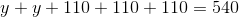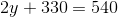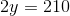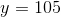The sum of the measures of a hexagon is. Therefore,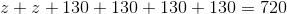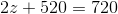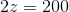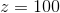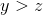, so (A) is greater.

### Example Question #7 : Hexagons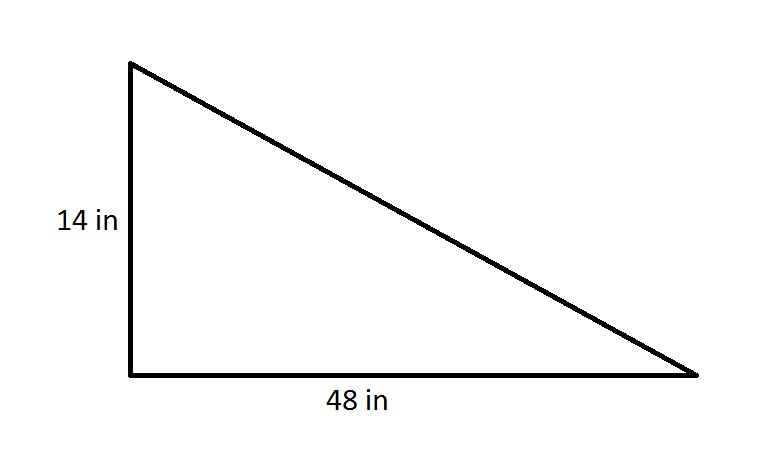A regular hexagon has the same perimeter as the above right triangle. What is the length of one side of the hexagon?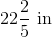The length cannot be determined from the information given.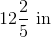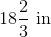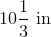Explanation:

By the Pythagorean Theorem, the hypotenuse of the right triangle is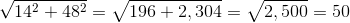inches, making its perimeter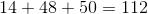inches.

The regular hexagon, which has six sides of equal length, has the same perimeter, so each side measures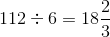inches.

### Example Question #8 : HexagonsA regular hexagon has the same perimeter as the above right triangle. What is the length of one side of the hexagon?The length cannot be determined from the information given.Explanation:

By the Pythagorean Theorem, the hypotenuse of the right triangle isinches, making its perimeterinches.

The regular hexagon, which has six sides of equal length, has the same perimeter, so each side measuresinches.